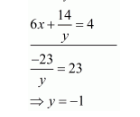# Solve the following systems of equations:

Question:

Solve the following systems of equations:

$2 x-\frac{3}{y}=9$

$3 x+\frac{7}{y}=2, y \neq 0$

Solution:

The given equations are:

$2 x-\frac{3}{y}=9 \ldots(i)$

$3 x+\frac{7}{y}=2 \ldots(i i)$

Multiply equation $(i)$ by 3 and $(i i)$ by 2 and subtract equation (ii) from (i) we get

$6 x-\frac{9}{y}=27$Put the value of $y$ in equation $(i)$, we get

$2 x-\frac{3}{-1}=9$

$\Rightarrow 2 x=6$

$\Rightarrow x=3$

Hence the value of $x=3$ and $y=-1$